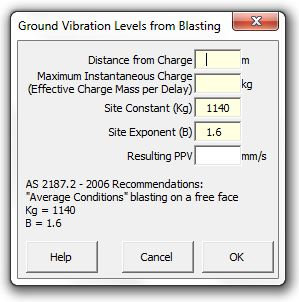### Strutt Help

Ground Vibration Levels from Blasting   1/1, 1/3

Strutt|Vibration|Blasting|Ground Vibration estimates the ground vibration expected from any particular blasting operations.Strutt implents the formula as:

V = K_g*(R/Q^(1//2))^(-B)

Where,
V is the ground vibration as vector peak particle velocity, mm/s
R is the distance between charge and point of measurement, m
Q is the maximum instantaneous charge (effective mass per delay), kg
K_g and B are constants related to site and rock properties for estimation purposes.

The constants K_g and B will vary from site to site. The Strutt default values of K_g = 1140 and B = 1.6 are the average conditions for blasting on a free face given in AS2187.2-2000.

Reference:

• AS2187.2-2006